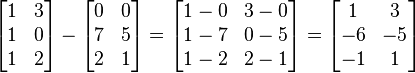## 3x3MATRIX Subtraction Calculator

Matrix 1 - Matrix 2
-
Result =
=

Subtract one matrix from another, as long as they have the same dimensions. A − B is computed by subtracting corresponding elements of A and B, and has the same dimensions as A and B. For example:Thinkcalculator.com provides you helpful and handy calculator resources.